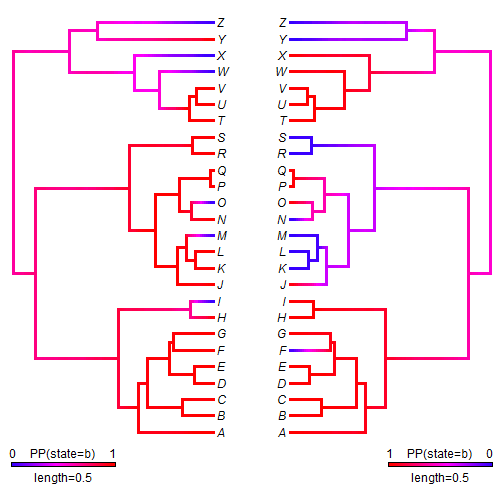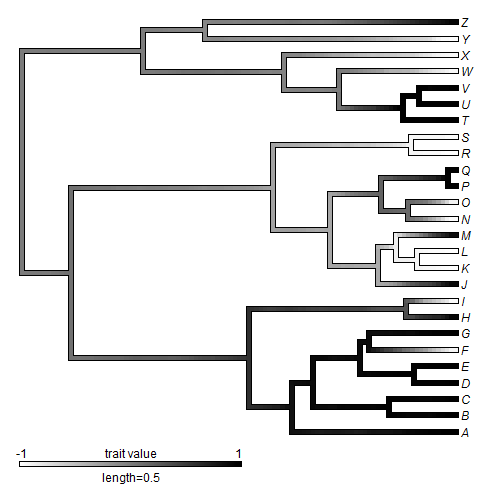## Wednesday, May 13, 2015

### Comparing two objects of class "densityMap"

A phytools user contacted me recently about comparing character reconstructions obtained using stochastic character mapping by the use of offset trees as in here. Although this, of course, can be done - I also have another suggestion. That is, to compare the correlation of two different `densityMap` style trees. A `densityMap` tree has the posterior density from stochastic mapping of a binary trait mapped finely on the edges and nodes of a phylogeny. For more information about this plotting method, just check out my blog.

The way we go about computing the correlation is by traversing the edges of the tree and computing the correlation as 1 if the probabilities of our character state are both 0 or both 1 on the two trees; -1 if the probability is 0 in one tree and 1 in the other; and somewhere in between for intermediate probabilities, depending on how high or low, and how similar, they are.

Here's a demo using simulated data:

``````## load packages
library(phytools)
## simulate a tree & two character data vectors
tree<-pbtree(n=26,tip.label=LETTERS,scale=1)
Q<-matrix(c(-1,1,1,-1),2,2)
colnames(Q)<-rownames(Q)<-letters[1:2]
x<-sim.history(tree,Q)\$states
``````
``````## Done simulation(s).
``````
``````x
``````
``````##   A   B   C   D   E   F   G   H   I   J   K   L   M   N   O   P   Q   R
## "b" "b" "b" "b" "b" "b" "b" "b" "a" "b" "b" "b" "a" "b" "a" "b" "b" "b"
##   S   T   U   V   W   X   Y   Z
## "b" "b" "b" "b" "a" "a" "b" "a"
``````
``````y<-sim.history(tree,Q)\$states
``````
``````## Done simulation(s).
``````
``````y
``````
``````##   A   B   C   D   E   F   G   H   I   J   K   L   M   N   O   P   Q   R
## "b" "b" "b" "b" "b" "a" "b" "b" "b" "b" "a" "a" "a" "a" "b" "b" "b" "a"
##   S   T   U   V   W   X   Y   Z
## "a" "b" "b" "b" "b" "b" "a" "a"
``````
``````## perform stochastic mapping
mx<-make.simmap(tree,x,nsim=100)
``````
``````## make.simmap is sampling character histories conditioned on the transition matrix
## Q =
``````
``````##           a         b
## a -1.258205  1.258205
## b  1.258205 -1.258205
``````
``````## (estimated using likelihood);
## and (mean) root node prior probabilities
## pi =
``````
``````##   a   b
## 0.5 0.5
``````
``````## Done.
``````
``````my<-make.simmap(tree,y,nsim=100)
``````
``````## make.simmap is sampling character histories conditioned on the transition matrix
## Q =
``````
``````##           a         b
## a -1.157145  1.157145
## b  1.157145 -1.157145
``````
``````## (estimated using likelihood);
## and (mean) root node prior probabilities
## pi =
``````
``````##   a   b
## 0.5 0.5
``````
``````## Done.
``````
``````## compute density maps
dmapx<-densityMap(mx,plot=FALSE)
``````
``````## sorry - this might take a while; please be patient
``````
``````dmapy<-densityMap(my,plot=FALSE)
``````
``````## sorry - this might take a while; please be patient
``````
``````## compare them visually
par(mfcol=c(1,2))
plot(dmapx)
plot(dmapy,direction="leftwards")
````````````## now compute the correlation between maps
obj<-dmapx
nl<-length(obj\$cols)-1
## here I recenter the probability on zero &
## compute the vector correlation
for(i in 1:length(obj\$tree\$maps)){
nx<-2*(as.numeric(names(dmapx\$tree\$maps[[i]]))-nl/2)/nl
ny<-2*(as.numeric(names(dmapy\$tree\$maps[[i]]))-nl/2)/nl
names(obj\$tree\$maps[[i]])<-round((nx*ny+1)/2*1000)
}
## change to an object of class "contMap"
obj\$lims<-c(-1,1)
class(obj)<-"contMap"
obj<-setMap(obj,c("white","black"))
par(mfcol=c(1,1))
plot(obj)
``````That's all there is to it. We see that regions of high certainty show up with high or low correlation - depending on the similarity between maps. Regions of low certainty will always show up with near zero correlation, which is precisely what we want.

That's it.